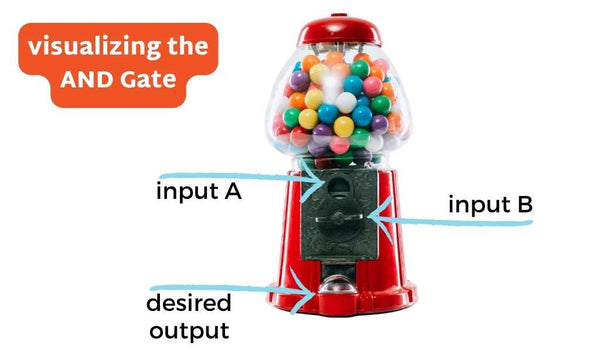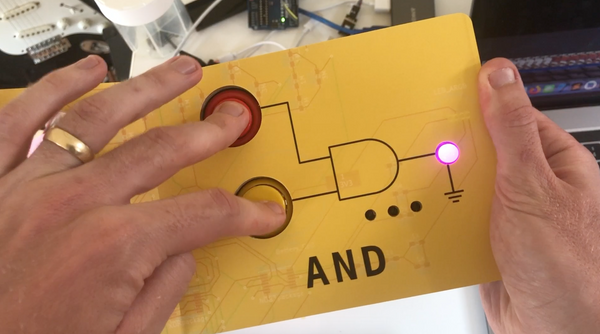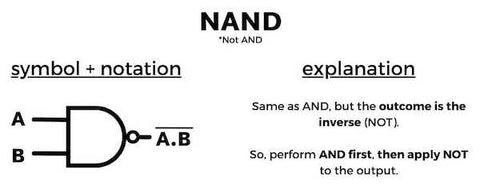# AND Gate | Tutorial with Examples, Truth Table, and Downloadable AssetsThe AND Gate is a digital logic gate that takes two or more inputs and produces a single output.

If you're a visual person, think of the AND gate like a gum ball machine. In order to dispense a gum ball, you have to A) insert your coin AND B) turn the lever.
- Input A and B are “ON”, you get 1 gum ball
- If either or all inputs, “OFF”, 0 gum balls for youThe And Gate is often used in combination with other logic gates to create more complex circuits and perform more intricate operations.

## The AND Gate

The AND gate is a digital logic gate that performs the logical operation on two or more binary inputs. The output of an AND gate is only HIGH (1) when all of its inputs are HIGH. If any of the inputs are LOW (0), then the output of the AND gate is also LOW (0).

*Fyi, you may see the following terms used interchangeably
1 | HIGH | ON
O | LOW | OFF

The Boolean expression given for an AND gate is that for Logical Multiplication, which is denoted by a single dot or full stop symbol ( . ) giving us the Boolean expression of:  Z = A.B.

## Truth Table of AND Gate

The truth table of an AND gate summarizes its behavior for all possible input combinations. The following table shows the truth table for a 2-input AND gate:## How Does an AND Gate Work?

The AND gate works by implementing the logical conjunction operation on its inputs.

In other words, it checks whether both or all inputs are HIGH, and if so, it produces a HIGH (1, ON) output. Otherwise, it produces a LOW (0, OFF) output. The AND gate can have any number of inputs, and the same logical conjunction operation applies to all of them.

How about some real world applications of AND Gates?

## Applications of AND Gates

Here’s how we applied an AND Gate to our book, Computer Engineering for Babies.AND gates have several applications in digital circuits. Some of the common applications are:

• Data transfer
• Push buttons
• Multiplexers
• Demultiplexers
• Counters
• Flip-flops
• Registers
• Data validation

## Different Types of AND Gates

There are different types of AND gates based on their input and output configurations. Some of the common types of AND gates are:

• 2-input AND gate
• 3-input AND gate
• 4-input AND gate
• 8-input AND gate
• NAND gate (complementary to AND gate)## Advantages of Using AND Gates

AND gates have several advantages in digital circuits. Some of the advantages are:

• They are simple to implement and understand.
• They have a wide range of applications in digital circuits.
• They can be combined with other logic gates to perform complex operations.
• They are easy to test and troubleshoot.

There are many ways to use a Gate in Computer Science and relating fields, here's how the AND Gate compares to the others.

## Comparing AND Gates with Other Logic Gates

AND OR NOT Gates may all seem similar, but there are some key differences that make them all unique and powerful in combination with each other.

AND gates are one of the fundamental types of logic gates used in digital circuits. They are used in combination with other logic gates to perform various operations. One of the most common ways to use AND gates is to chain them together to create logic circuits. Logic circuits enable computers to perform more complex operations than they could with just a single gate.

One key difference between AND gates and other logic gates is their output. The output of an AND gate is only high (1) when all of its inputs are high. Other logic gates, such as the OR gate, XOR Gate, and NOT gate, have different output characteristics. OR gates produce a high output when at least one of their inputs is high, while NOT gates produce a high output when their input is low (0).

Another important difference between AND gates and other logic gates is their use in Boolean algebra. AND gates correspond to the Boolean operation of multiplication. This means that when two Boolean variables are multiplied together, the result is 1 only if both variables are 1. OR gates, on the other hand, correspond to addition in Boolean algebra, while NOT gates correspond to inversion or negation of a Boolean variable.

## The AND Gate in Digital Logic | Final Thoughts

In summary, AND gates have a unique output characteristic and correspond to multiplication in Boolean algebra. They are often used in combination with other logic gates to create logic circuits that perform complex operations. Other logic gates, such as OR gates and NOT gates, have different output characteristics and correspond to different Boolean operations.